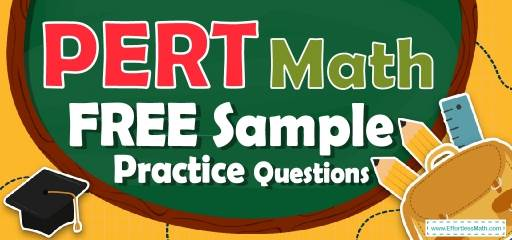# PERT Math FREE Sample Practice QuestionsPreparing for the PERT Math test? To succeed on the PERT Math test, you need to practice as many real PERT Math questions as possible.  There’s nothing like working on PERT Math sample questions to measure your exam readiness and put you more at ease when taking the PERT Math test. The sample math questions you’ll find here are brief samples designed to give you the insights you need to be as prepared as possible for your PERT Math test.

Check out our sample PERT Math practice questions to find out what areas you need to practice more before taking the PERT Math test!

Start preparing for the 2022 PERT Math test with our free sample practice questions. Also, make sure to follow some of the related links at the bottom of this post to get a better idea of what kind of mathematics questions you need to practice.

## 10 Sample PERT Math Practice Questions

1- Solve the equation: $$log_{4⁡}(x+2) – log_4⁡(x-2) = 1$$

A. 10

B. $$\frac{3}{10}$$

C. $$\frac{10}{3}$$

D. 3

2- Solve: $$e^{(5x + 1 )}= 10$$

A. $$\frac{ln⁡(10) + 1}{5}$$

B. $$\frac{ln⁡(10) – 1}{5}$$

C. $$5ln (10) + 2$$

D. $$5ln (10) – 2$$

3- If $$f(x) = x – \frac{5}{3}$$ and $$f ^{–1}$$ is the inverse of $$f(x)$$, what is the value of $$f ^{–1(5)$$?

A. $$\frac{10}{3}$$

B. $$\frac{3}{20}$$

C. $$\frac{20}{3}$$

D. $$\frac{3}{10}$$

4- What is cos $$30^{\circ}$$?

A. $$\frac{1}{2}$$

B. $$\frac{{\sqrt{2}}}{2}$$

C. $$\frac{{\sqrt{3}}}{2}$$

D. $$\sqrt{3}$$

5- If $$\theta$$ is an acute angle and sin $$\theta = \frac{3}{5}$$, then cos $$\theta$$ =?

A. $$-1$$

B. 0

C. $$\frac{4}{5}$$

D. $$\frac{5}{4}$$

6- What is the solution of the following system of equations?
$$-2x- y = -9$$
$$5x-2y= 18$$

A. $$(–1, 2)$$

B. $$(4, 1)$$

C. $$(1, 4)$$

D. $$(4, -2)$$

7- Solve.
$$|9 – (12 ÷ | 2 – 5 |)| =$$?

A. 9

B. $$-6$$

C. 5

D. $$-5$$

8- If $$log_{2⁡}x = 5$$, then $$x =$$?

A. $$2^{10}$$

B. $$\frac{5}{2}$$

C. $$2^{6}$$

D. 32

9- What’s the reciprocal of $$\frac{x^3}{16}$$?

A. $$\frac{16}{x^3}-1$$

B. $$\frac{48}{x^3}$$

C. $$\frac{16}{x^3}+1$$

D. $$\frac{16}{x^3}$$

10- Find the inverse function for $$ln (2x + 1)$$?

A. $$\frac{1}{2}(e^{x }– 1)$$

B. $$(e^{x }+ 1)$$

C. $$\frac{1}{2}(e^{x }+ 1)$$

D. $$(e^{x }– 1)$$

## Best PERT Math Prep Resource for 2022

1- C
METHOD ONE
$$log_4⁡(x+2) – log_4⁡(x-2) = 1$$
Add $$log_4⁡(x-2)$$ to both sides
$$log_4⁡(x+2) – log_4⁡(x-2)+ log_4⁡(x-2)= 1 + log_4⁡(x-2)$$
$$log_4⁡(x+2) = 1 + log_4⁡(x-2)$$
Apply logarithm rule:
$$a = log_b⁡(b^a) ⇒ 1 = log_4⁡(4^1) = log_4⁡(4)$$
then: $$log_4⁡(x+2) = log_4⁡(4) + log_4⁡(x-2)$$
Logarithm rule: $$log_c⁡(a) + log_c⁡(b) = log_c⁡(ab)$$
then: $$log_4⁡(4) + log_4⁡(x-2) = log_4⁡(4(x-2))$$
$$log_4⁡(x+2) = log_4⁡(4(x-2))$$
When the logs have the same base:
$$log_b⁡(f(x)) = log_b⁡(g(x)) ⇒ f(x) = g(x) (x+2) = 4(x-2)$$
$$x = \frac{10}{3}$$

METHOD TWO
We know that:
$$log_a⁡b-log_a⁡c=log_a\frac{b}{c}⁡\space and \space log_a⁡b=c⇒b=a^c$$
Then: $$log_4⁡(x+2)- log_4⁡(x-2)=log_4\frac{(x + 2)}{(x – 2)}⁡=1⇒\frac{(x + 2)}{(x – 2)}=4^1=4⇒x+2=4(x-2) ⇒x+2=4x-8⇒4x-x=8+2→3x=10⇒x=\frac{10}{3}$$

2- B
$$e^{(5x + 1 )}= 10$$
If $$f(x) = g(x)$$, then $$ln(f(x)) = ln(g(x))$$
$$ln⁡(e^{(5x + 1 )})= ln(10)$$
Apply logarithm rule:
$$log_a⁡(x^b) = b log_a⁡(x)$$
$$ln⁡(e^{(5x + 1 )})= (5x + 1)ln(e)$$
$$(5x + 1)ln(e) = ln(10)$$
$$(5x + 1)ln(e) = (5x + 1)$$
$$(5x + 1) = ln(10)$$
$$⇒x = \frac{ln⁡(10) – 1}{5}$$

3- C
$$f(x) = x –\frac{5}{3}⇒ y = x – \frac{5}{3}⇒ y+ \frac{5}{3}=x$$
$$f^{-1 }= x+ \frac{5}{3}$$
$$f ^{–1}(5) = 5 +\frac{5}{3}=\frac{20}{3}$$

4- C
cos $$30^{\circ} = \frac{\sqrt 3}{2}$$

5- C
sin$$θ=\frac{3}{5}⇒$$ we have following triangle, then:
$$c=\sqrt {(5^2-3^2 )}=\sqrt{(25-9)}=\sqrt 16=4$$
cos$$θ=\frac{4}{5}$$

6- B
$$-2x- y = -9$$
$$5x-2y= 18$$
$$⇒$$ Multiplication $$(–2)$$ in first equation
$$4x +2y =18$$
$$5x-2y= 18$$
Add two equations together $$⇒ 9x =36 ⇒ x= 4$$ then: $$y = 1$$

7- C
$$|9 – (12 ÷ | 2 – 5 |)| = |(9-(12÷|-3|))|=|9-(12÷3)|=|9-4|=|5|=5$$

8- D
METHOD ONE
$$log_{2}⁡x = 5$$
Apply logarithm rule: $$a = log_b⁡(b^a)$$
$$5 = log_2⁡(2^5) = log_2⁡(32)$$
$$log_2⁡x = log_2⁡(32)$$
When the logs have the same base: $$log_b⁡(f(x)) = log_b⁡(g(x))⇒ f(x) = g(x)$$
then: $$x = 32$$

METHOD TWO
We know that:
$$log_a⁡b=c⇒b=a^c$$
$$log_2⁡x=5⇒x=2^5=32$$

9- D
$$\frac{x^3}{16}$$
$$⇒$$ reciprocal is : $$\frac{16}{x^3}$$

10- A
$$f(x) = ln (2x + 1)$$
$$y = ln (2x + 1)$$
Change variables $$x$$ and $$y: x = ln (2y + 1)$$
solve: $$x = ln (2y + 1)$$
$$y = \frac{e^{x}-1}{2}=\frac{1}{2}(e^{x} – 1)$$

## More from Effortless Math for PERT Test …

### Looking for a PERT math study guide to help you prepare for your PERT Math test?

Top 5 PERT Math Study Guides will introduce you to the books for the PERT Math test!

### Do you want to get your desired result in the PERT math test?

Do not forget to follow the important tips of the test day!: PERT Math – Test Day Tips.

### Can’t handle the difficult math questions on the PERT test?

The best way to handle this is by reviewing the Top 10 PERT Math Practice Questions.

## Have any questions about the PERT Test?

### What people say about "PERT Math FREE Sample Practice Questions - Effortless Math: We Help Students Learn to LOVE Mathematics"?

No one replied yet.

X
49% OFF

Limited time only!

Save Over 49%

SAVE $34 It was$69.99 now it is \$35.99